Power in the voltage dividerCustom SearchMultiple-load voltage dividersMatter, Energy, and ElectricityVoltage divider with positive and negative voltage requirementsPOWER IN THE VOLTAGE DIVIDER
Power in the voltage divider is an extremely important quantity.
The power dissipated by the resistors in the voltage divider should be calculated to determine the power handling requirements of the resistors. Total power of the circuit is needed to determine the power requirement of the source.

The power for the circuit shown in figure 3-65 is calculated as follows:

Given: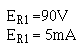Solution: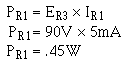The power in each resistor is calculated just as for R1. When the calculations are performed, the following results are obtained:To calculate the power for load 1:

Given: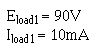Solution: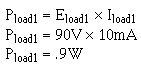The power in each load is calculated just as for load 1. When the calculations are performed, the following results are obtained.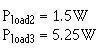Total power is calculated by summing the power consumed by the loads and the power dissipated by the divider resistors. The total power in the circuit is 15.675 watts.

The power used by the loads and divider resistors is supplied by the source. This applies to all electrical circuits; power for all components is supplied by the source.

Since power is the product of voltage and current, the power supplied by the source is equal to the source voltage multiplied by the total circui current (Es x IT).

In the circuit of figure 3-65, the total power can be calculated by:

Given:Solution:Integrated Publishing, Inc. - A (SDVOSB) Service Disabled Veteran Owned Small Business